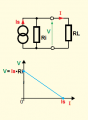# Non-ideal voltage source V-I characteristic

#### Xefro1337

Joined Aug 18, 2019
2

#### MrChips

Joined Oct 2, 2009
21,041
Welcome to AAC!

R is a linear component that obeys Ohm's Law:

I = V / R

What does this relationship look like when drawn on the V - I graph?

#### ci139

Joined Jul 11, 2016
1,441
as how i('m able to) get it the "Non-ideal VS***" is a regulated PSU type of (so that's a bit modified https://en.wikipedia.org/wiki/Internal_resistance)

. . . so if you attach the TEST current sink in ?series/?parallel with it (& that without the R.LOAD) you see the U(I) graph*** on it's terminals . . .

#### Xefro1337

Joined Aug 18, 2019
2
Welcome to AAC!

R is a linear component that obeys Ohm's Law:

I = V / R

What does this relationship look like when drawn on the V - I graph?
I just cannot seem to grasp the relationship of the resistor in respect to the V-I graph and finding the current with the resistor value.

#### Jony130

Joined Feb 17, 2009
5,162
Here you have an example of how to plot a 1Ω resistance into your V-I graph.All you need is a Ohm's law.

1A*1Ω = 1V
2A*1Ω = 2V
3A*1Ω = 3V

And plot in on the graph.

But we can find is analytics.

Simply notice tha that until load current is smaller than 2.5A. Your Non-ideal voltage source act just like an ideal voltage source.

But for a large currents (I > 2.5A) your Non-ideal voltage source act lika a non-ideal current source with internal resistance.

As I show here:Now you can use your V-I graph and solve for Ri resistance and read from the graph the Is value.

Last edited:

#### Danko

Joined Nov 22, 2017
927

#### WBahn

Joined Mar 31, 2012
25,731